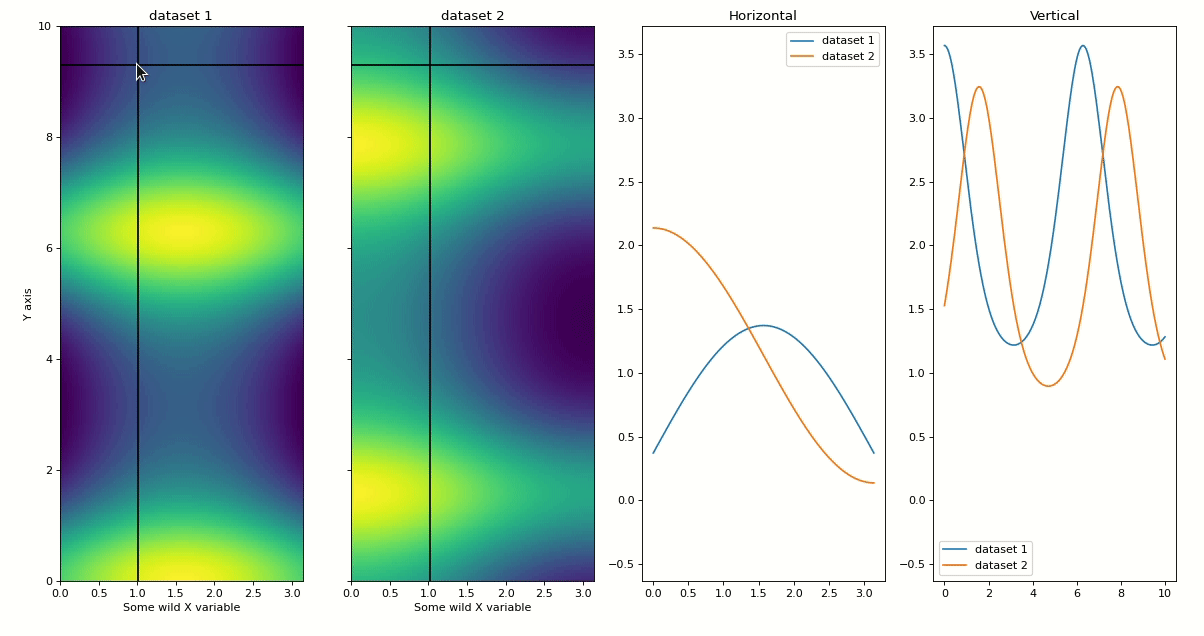This page was generated from examples/heatmap-slicer.ipynb. Interactive online version: (Warning: The interactions will be much laggier on Binder than on your computer.)

# Heatmap Slicer¶

[ ]:

%matplotlib widget
import matplotlib.pyplot as plt
import numpy as np

from mpl_interactions import heatmap_slicer


## Comparing heatmaps¶

Sometimes I find myself wanting to compare horizontal or vertical slices across two different heatmaps with the same shape. The function heatmap_slicer makes this easy and should work for any number of heatmaps from 1 to many (likely not all the way $$\inf$$ though).

The most important options to play with are slices = {'both', 'vertical', 'horizontal'}, and interaction_type = {'move', 'click'}

[ ]:

x = np.linspace(0, np.pi, 100)
y = np.linspace(0, 10, 200)
X, Y = np.meshgrid(x, y)
data1 = np.sin(X) + np.exp(np.cos(Y))
data2 = np.cos(X) + np.exp(np.sin(Y))
fig, axes = heatmap_slicer(
x,
y,
(data1, data2),
slices="both",
heatmap_names=("dataset 1", "dataset 2"),
labels=("Some wild X variable", "Y axis"),
interaction_type="move",
)[ ]: